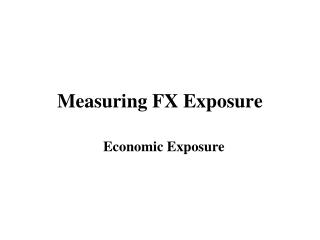Download PresentationMeasuring FX Exposure

# Measuring FX Exposure

Download Presentation## Measuring FX Exposure

- - - - - - - - - - - - - - - - - - - - - - - - - - - E N D - - - - - - - - - - - - - - - - - - - - - - - - - - -
##### Presentation Transcript

1. Measuring FX Exposure Economic Exposure

2. Economic Exposure • Economic exposure (EE): EE measures how an unexpected change in St affect the future cash flows of the firm. • • Economic exposure is: Subjective. • Difficult to measure. • • Measuring CFs. • We can use accounting data (changes in EAT) or financial/economic data (stock returns) to measure EE. Economists tend to like more non-accounting data measures. • Note: Since St is very difficult to forecast, the actual change in St (ef,t) can be considered “unexpected.”

3. Measuring Economic Exposure • An Easy Measure of EE Based on Financial Data • We can easily measure how CF and St move together: correlation. • Example: Kellogg’s and IBM’s EE. • Using monthly stock returns for Kellogg’s (Krett) and monthly changes in St (USD/EUR) from 1/1994-2/2008, we estimate ρK,s (correlation between Krett and st) = 0.154. • It looks small, but away from zero. We do the same exercise for IBM, obtaining ρIBM,s=0.056, small and close to zero. ¶ • Better measure: 1) Run a regression on CF against (unexpected) St. 2) Check statistical significance of regression coeff’s.

4. Testing and Evaluating EE with a regression • Steps: • (1) Collect data on CF and St (available from the firm's past) • (2) Estimate the regression: CFt =  + ß St + t, •  ß measures the sensitivity of CF to changes in St. •  the higher ß, the greater the impact of St on CF. • (3) Test for EE  H0 (no EE): β = 0 • H1 (EE): β ≠ 0 • (4) Evaluation of this regression: t-statistic of ß and R2. • Rule: |tβ= ß/SE(ß)| > 1.96 => ß is significantly different than zero at 5% level. • • For companies listed on exchanges, we can use stock prices instead of CFs & stock returns instead of changes in CFs.

5. Example: Kellogg’s EE. • Now, using the data from the previous example, we run the regression: Krett =  + ß ef,t + t • R2 = 0.023717 • Standard Error = 0.05944 • Observations = 169 • Coefficients Standard Error t-Stat P-value • Intercept (α) 0.003991 0.004637 0.860756 0.390607 • ef,t (β) 0.551059 0.273589 2.014185 0.045595 • Analysis: • We reject H0, since |tβ = 2.01| > 1.96 (significantly different than zero). • Note, however, that the R2 is very low! (The variability of st explains less than 2.4% of the variability of Kellogg’s returns.) ¶

6. Example: IBM’s EE. • Now, using the IBM data, we run the regression: • IBMrett =  + ß ef,t + t • R2 = 0.003102 • Standard Error = 0.09462 • Observations = 169 •   Coefficients Standard Error t-Stat P-value • Intercept (α) 0.016283 0.007297 2.231439 0.026983 • ef,t (β) -0.20322 0.2819 -0.72089 0.471986 • Analysis: • We cannot reject H0, since |tβ = -0.72| < 1.96 (not significantly different than zero). • Again, the R2 is very low. (The variability of st explains less than 0.3% of the variability of IBM’s returns.) ¶

7. • Sometimes the impact of St is not felt immediately by a firm. •  contracts and short-run costs (short-term adjustment difficult). • Example: For an exporting U.S. company a sudden appreciation of the USD increases CF in the short term. • Run a modified regression: • CFt =  + ß0St + ß1St-1 + ß2St-2 + ß3St-3 + ... + t. • The sum of the ßs measures the sensitivity of CF to St. • Practical issue: Number of lags? • Usual practice: Include at most two years of information.

8. Example: HAL runs the following regression. • CFt = .456 + .421 St + .251 St-1 +.052 St-2. R2= .168. • (.89) (2.79) (2.01) (0.77) • HAL's HKD CF (in USD) sensitivity to St is 0.672 (.421+.251). a 1% appreciation of the HKD will increase HKD CF (translated into USD) by 0.672%. ¶ • Evidence: For large companies (MNCs, Fortune 500),  is not significantly different than zero. We cannot reject Ho: No EE.

9. A Measure Based on Accounting Data • It requires to estimate the net cash flows of the firm (EAT or EBT) under several FX scenarios. (Easy with an excel spreadsheet.) • Example: IBM HK provides the following info: • Sales and cost of goods are dependent on St • St = 7 HKD/USD St = 7.70 HKD/USD • Sales (in HKD) 300M 400M • Cost of goods (in HKD) 150M200M • Gross profits (in HKD) 150M 200M • Interest expense (in HKD) 20M20M • EBT (in HKD) 130M180M • EBT (in USD at St=7) : HKD 130M/7 HKD/USD = USD 18.57M • EBT (in USD at St = 7.7): HKD 180M/7.70 HKD/USD = USD 23.38M

10. Example (continuation): • A 10% depreciation of the HKD, increases the HKD cash flows from HKD 130M to HKD 180M, and the USD cash flows from USD 18.57M to USD 23.38. • Q: Is EE significant? • A: We can calculate the (pseudo) elasticity of CF to changes in St. • For example, in USD, a 10% depreciation of the HKD produces a change of 25.9% in EBT. Quite significant. But you should note that the change in exposure is USD 4.81M. • This amount might not be significant for IBM! (Judgment call needed.) ¶ • Note: Obviously, firms will simulate many scenarios to gauge the sensitivity of EBT to changes in exchange rates.